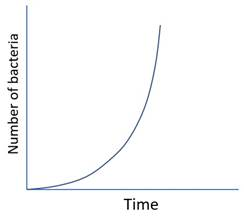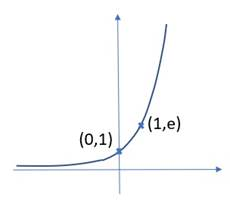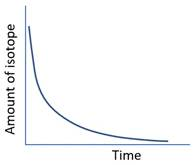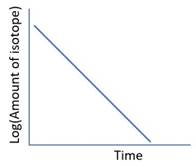# 2012B13 Using an example of exponential decay, define and outline the important features of an exponential function.

### List:

·     Exponential function

·     Natural exponential function

·     Exponential decay

### Exponential function:

 Equation ·   y = ax ·   Output equals a constant to the power of the input Graph Runaway curve### Natural exponential function:

 Equation ·   y=Aex = δy/δx (where e = 2.718281828…) ·   i.e. rate of change of Y is proportional to amount of Y GraphsUse Used to model time-dependent exponential processes ·   Drug metabolism ·   Alveolar filling ·   Pre-oxygenation ·   Radioactive decay

### Exponential decay:

 Equation N = N0e-kt Where ·   N = amount of isotope ·   N0 = amount of isotope at t=0 GraphRate constant (k) ·     Proportion of isotopes decaying per unit time, had the initial rate of decay continued ·   Units: min-1 Time constant (τ) ·   Time taken for completion had the initial rate of decay continued ·   τ = 1/k ·   Units: minutes ·   ~95% completion at 3 x τ Half life (t1/2) ·   Time taken for 50% decay ·   t1/2 = τ x loge2 ·   ~97% completion =  5 x t1/2

Feedback welcome at ketaminenightmares@gmail.com